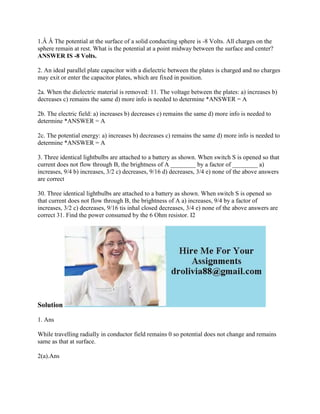Ce diaporama a bien été signalé.
Le téléchargement de votre SlideShare est en cours. ×

# 1-The potential at the surface of a solid conducting sphere is -8 Volt.docx

Publicité
Publicité
Publicité
Publicité
Publicité
Publicité
Publicité
Publicité
Publicité
Publicité
PublicitéChargement dans…3
×

## Consultez-les par la suite

1 sur 2 Publicité

# 1-The potential at the surface of a solid conducting sphere is -8 Volt.docx

1.Â Â The potential at the surface of a solid conducting sphere is -8 Volts. All charges on the sphere remain at rest. What is the potential at a point midway between the surface and center? ANSWER IS -8 Volts.
2. An ideal parallel plate capacitor with a dielectric between the plates is charged and no charges may exit or enter the capacitor plates, which are fixed in position.
2a. When the dielectric material is removed: 11. The voltage between the plates: a) increases b) decreases c) remains the same d) more info is needed to determine *ANSWER = A
2b. The electric field: a) increases b) decreases c) remains the same d) more info is needed to determine *ANSWER = A
2c. The potential energy: a) increases b) decreases c) remains the same d) more info is needed to determine *ANSWER = A
3. Three identical lightbulbs are attached to a battery as shown. When switch S is opened so that current does not flow through B, the brightness of A ________ by a factor of ________ a) increases, 9/4 b) increases, 3/2 c) decreases, 9/16 d) decreases, 3/4 e) none of the above answers are correct
30. Three identical lightbulbs are attached to a battery as shown. When switch S is opened so that current does not flow through B, the brightness of A a) increases, 9/4 by a factor of increases, 3/2 c) decreases, 9/16 tis inhal closed decreases, 3/4 e) none of the above answers are correct 31. Find the power consumed by the 6 Ohm resistor. I2
Solution
1. Ans
While travelling radially in conductor field remains 0 so potential does not change and remains same as that at surface.
2(a).Ans
we know that potential across a capacitor is given by V = Q/C. When we will remove dielectric C decreases so potential difference across capacitor increases.
2(b) Ans
As potential difference across capacitor increases and dielectric is removed so electric field given by E=V/d increases.
2(c) Ans
We know that potential energy of capacitor having charge Q is given by U = (Q^2)/2C. So when dielectric is removed C decreases so U increases.
.

1.Â Â The potential at the surface of a solid conducting sphere is -8 Volts. All charges on the sphere remain at rest. What is the potential at a point midway between the surface and center? ANSWER IS -8 Volts.
2. An ideal parallel plate capacitor with a dielectric between the plates is charged and no charges may exit or enter the capacitor plates, which are fixed in position.
2a. When the dielectric material is removed: 11. The voltage between the plates: a) increases b) decreases c) remains the same d) more info is needed to determine *ANSWER = A
2b. The electric field: a) increases b) decreases c) remains the same d) more info is needed to determine *ANSWER = A
2c. The potential energy: a) increases b) decreases c) remains the same d) more info is needed to determine *ANSWER = A
3. Three identical lightbulbs are attached to a battery as shown. When switch S is opened so that current does not flow through B, the brightness of A ________ by a factor of ________ a) increases, 9/4 b) increases, 3/2 c) decreases, 9/16 d) decreases, 3/4 e) none of the above answers are correct
30. Three identical lightbulbs are attached to a battery as shown. When switch S is opened so that current does not flow through B, the brightness of A a) increases, 9/4 by a factor of increases, 3/2 c) decreases, 9/16 tis inhal closed decreases, 3/4 e) none of the above answers are correct 31. Find the power consumed by the 6 Ohm resistor. I2
Solution
1. Ans
While travelling radially in conductor field remains 0 so potential does not change and remains same as that at surface.
2(a).Ans
we know that potential across a capacitor is given by V = Q/C. When we will remove dielectric C decreases so potential difference across capacitor increases.
2(b) Ans
As potential difference across capacitor increases and dielectric is removed so electric field given by E=V/d increases.
2(c) Ans
We know that potential energy of capacitor having charge Q is given by U = (Q^2)/2C. So when dielectric is removed C decreases so U increases.
.

Publicité
Publicité